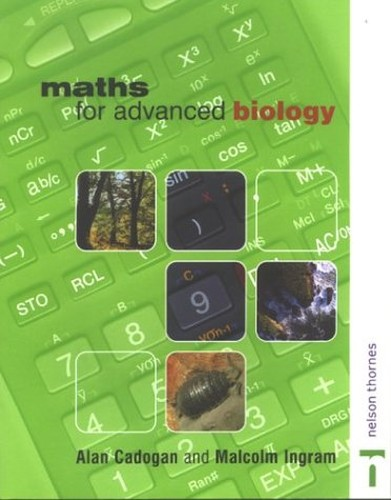Free Shipping in Australia
Proud to be B-Corp\$17.99
Condition - Very Good
Only 3 left

## Summary

Written by teachers and covering the Maths requirements of the specifications, this text is meant for classroom work and homework exercises. Meant for AS and A2 Levels, it aims to provide explanations of the mathematical concepts and how these are applied in Biology. It includes worked examples and examination style questions and answer sections.

## Maths for Advanced Biology Summary

Relevant for AS and A2 Levels of study and designed to be accessible and friendly in format, the text is aimed at providing clear and concise explanations of the mathematical concepts and how these are then applied in Biology. Worked examples are included throughout encouraging students to grasp the subject matter with ease. Examination style questions and answer sections provide the ideal opportunity for continuous progression and to consolidate learning.

Part 1 Numbers - Egypt to metric: numbers and the biologist; basic forms of notation; rounding and significant figures; using a scientific calculator; use of tables in biology; exam questions; answers to test yourself questions. Part 2 Symbols and units - the Greeks have a word for it: words and the biologist; using symbols for size in biology; using scales and measuring biological objects; using units and symbols; units; exam questions; answers to test yourself questions. Part 3 Algebra - x - the unknown: equations and rules; equations and ecology; the Hardy-Weinberg equilibrium; exam questions; answers to test yourself questions. Part 4 Indices, powers of ten, scientific notation: indices; powers of ten; scientific notation (or standard form); exam questions; answers to test yourself questions. Part 5 Ratios and getting things in proportion: ratios; the use of ratios in genetics; ratios in other biological examples; exam questions; answers to test yourself questions. Part 6 Displaying data -graphs, charts and scales: dealing with data; drawing graphs; plotting and drawing line graphs; other types of graph; exam questions; answers to test yourself questions.

GOR002203329
9780748765065
0748765069